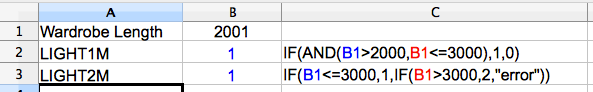# Calc (Openoffice / Libreoffice) If statements using 'between' or 'and'

Posted on Sat 15 December 2012 in Business, WebDev & Code

It's always annoyed me when I want to write an if statement where true is based upon more than one fact and I have to nest if statements. Today I looked at lots of other forumulas and found one that let me create an if_between effect. Like "IF a number is between 2000 and 3000 DO this ELSE that"

## First, a little background on simple if statements

An if statement in calc is written:

```=if(test_something,do_if_thats_true,do_if_thats_false)
```

test_something has to return true or false, so we can have if we have cell A1 with value of 6, A1=6 would return true whereas A1=5 would return false and A1="six" would return false (the written six is not the same as the number 6).

If our test_something is true, calculate the do_if_thats_true section. If our test_something returns false, then calculate the do_if_thats_false section.

Example; if we put this formula in cell B1

```=if(A1=6,"I see the number 6","Not 6")
```

then put '6' inside A1, B1 is displayed as "I see the number 6"

if we put anything else inside A1 ('six',7,true,false,or even leave it empty) then B1 is displayed as "Not 6"

## Using 'between' or 'and' in our if statements

That first example was easy. I often find myself wanting to do something more clever than that though. Here's a real example I've just had to figure out for my work. We sell bedroom wardrobes and I'm creating a spreadsheet that given a particular width will work out which component parts we need to order.

I need to order a different number of lights depending on the width of the wardrobe. My lights come in strips of 1 metre or 2 metre lengths that can plug together and be cut to fit shorter lengths.  My wardrobes can be between 1.2m and 4m long.  Therefore:

• if my wardrobe is 2m or less I need a single 2m length of light.
• if my wardrobe is more than 2m but less than 3m I need a 2m and a 1m length of light
• if my wardrobe is more than 3m i need 2 x 2m lengths of light.

I have two different product codes, let us call them 'LIGHT1M' and 'LIGHT2M' and I need my spreadsheet to tell the user how many of each code to order for any length of wardrobe the customer has.

So I create a spreadsheet that needs to one input from the user; the Wardrobe Length. I then have a row for each component. In column B I can calculate the quantity of the components needed from the wardrobe length. I've also copied my forumula to column C so you can see it (it is displayed because I haven't put the = sign in front).

My results in column B are blue because I always colour calculated results blue so I and users know not to change the field manually.In B3 (the easier part) I nest two if statements. If  "length is less than or equal to 3000", then I need a single LIGHT2M so I can output the number 1. If my length is more than 3000 I'll move into the 'false' part of the if statement. Here I've put another if statement (and you can nest if statements quite a long way - I think I've had seven in a row before - but it gets confusing so sometimes it makes sense to put each statement in a separate cell calculating based on the result of another cell) and that says if my "length is more than 3000" I want to return the number 2 as my quantity to order.  If I get here and my length is less than 3000 I return error. With my code here that can never happen. However I do this because I make mistakes (frequently!). If you'd like an example of an easy mistake to make, change B3 to read "if(B3\<3000..." [remove the = from the \<=] and put the wardrobe width as 3000 and see what happens. If I'm writing something that should never result in false (or true), I often output a text description to tell me where the error is.

## Now the interesting bit

There is a forumla called 'and' which works like this:

```=and(something_that_returns_true, something_else_that_returns_true, ...repeat other statements as you need...)
```

if every 'something_that_returns_true' in our and statement returns true, the result is true. I don't think there is a limit to how many statements we can include in this but I've not pushed it very far. If one of them returns FALSE, our result is FALSE

In my case, I need a statement that will work as ifbetween(something), and I get the same effect by making two tests:

• is my wardrobe greater than 2000?
• is my wardrobe less than or equal to 3000?

Providing both of those are true, and(...) returns true to my if statement. So if both of those are true, I can return the value 1 as I need a 1m length of light if my wardrobe is between 2m and 3m long (and I realise I'm switching units, 1 metre = 1000 mm and I've been using mm in my spreadsheet  - sorry if that's confusing!). If either of those tests were false, and(...) returns false and my if statement returns 0.

## One last note:

If statements are using True and False.  the number 1 is also the value of True and the number 0 is also the value of False. There's nothing to stop you confusing yourself by doing something like 'if(something_is_true,0,1)' then setting the cell display to 'boolean' [menu > formatting > cells > numbers] so when the result is true you actually see the result false!